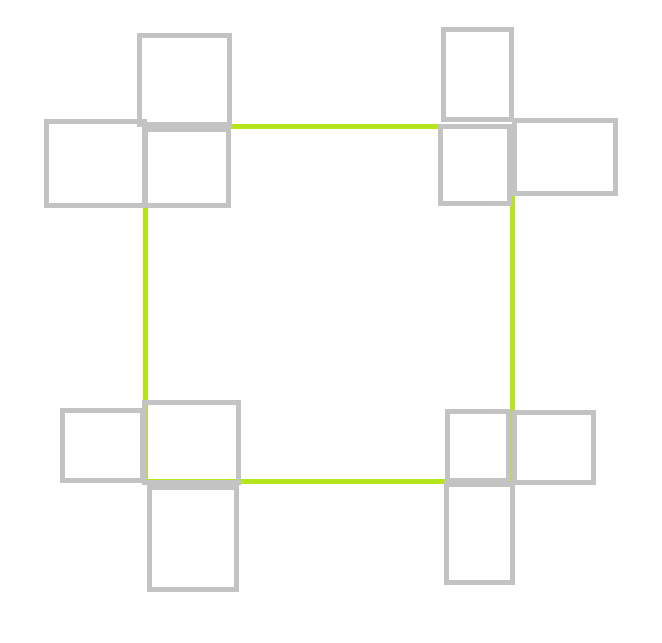# sparse-table算法

## 区间最值

st算法可以用于计算区间最值，不同于其它区间最值查询算法，st算法单次查询的时间复杂度仅为$O(1)$。下面讲解st算法。

$f(i,j)=min(f(i,j-1),f(i+2^{j-1},j-1))$

## LCA

LCA问题可以转换为区间最值问题，之后利用st算法就可以高效求解了。

void dfs(root){
root.enter = list.size();
list.add(root);
for(Node child : root.children)
{
dfs(child);
list.add(root);
}
root.leave = list.size() - 1;
}


1. 区间$I$中一定包含$u$与$v$的$x$。
2. $x$是区间$I$中拥有最小$enter$值的结点。

## 二维ST

ST可以直接推广到二维空间，记st[i][j][k][t]表示$x\in [i,i+2^j)$，$y\in [k,k+2^t)$的最值。因此对于大小为$n\times m$预处理的时间复杂度为$O(nm\log_2n\log_2m)$。查询实际上是查询4个矩形的并集，因此时间复杂度是$O(1)$。

# 高维莫队

• 普通的序列上的莫队，请求由$l,r$两个属性决定，我们可以将其映射到二维空间上。现在我们从$0$出发，要处理所有请求（经过所有请求），莫队算法提供的步骤数为$O(2N^{1.5})$。这里每一步的费用为1，因此时间复杂度也是$O(2N^{1.5})$。
• 考虑在二维数组上，我们需要查询某个子矩阵中的各种信息。每个请求可以由$l,r,b,t$决定，可以映射到四维空间。因此用莫队解决所需步骤数为$O(4N^{1.75})$。当然你说这时间复杂度怎么可能，当然我们这里并没有提及时间复杂度，实际上二维数组上每移动一步的费用为$O(N)$(比如$l$增大，对应要删除最左边的列，共$O(N)$个元素），因此时间复杂度为$O(4N^{2.75})$，依旧比暴力的$O(N^3)$好。
• 考虑带修改莫队，每个请求都由$l,r,time$所决定，因此可以映射到三维空间。所以用莫队解决所需步骤数为$O(3N^{1.667})$。但是和二维数组上的莫队不同，这里每步的费用也是$O(1)$，因此时间复杂度为$O(3N^{1.667})$。

# 一类阈值查询问题

1. 给定区间$l_i,r_i,x_i$，对于$l_i<j<r_i$，将$a_j$修改为$\max(a_j,x_i)$
2. 查询区间$l_i,r_i,x_i$，输出$\sum_{j=l}^r\max(a_j-x_i,0)$

1. 给定区间$l_i,r_i,x_i$，对于$l_i<j<r_i$，将$a_j$修改为$\max(a_j,x_i)$
2. 查询区间$l_i,r_i,x_i$，输出$\sum_{j=l}^r\max(a_j-x_i,0)$

# 多维平面操作问题# 双区间操作问题

1. 给定$l,r$，操作$a_l,a_{l+1},\ldots,a_r$。
2. 给定$l,r$，操作$a_{P_l},a_{P_{l+1}},\ldots,a_{p_r}$。

# 区间k小问题

1. 给定$l,r,k$，找到$a_l,a_{l+1},\ldots,a_r$中第k小的数。

1. 给定$l,r,k$，找到$a_l,a_{l+1},\ldots,a_r$中第k小的数。
2. 给定$i,x$，将$a_i$修改为$x$

1. 给定$l,r,k$，找到$a_l,a_{l+1},\ldots,a_r$中第k小的数。
2. 给定$l,r,x$，将$a_l,a_{l+1},\ldots,a_r$修改为$x$

1. 给定$u,v,k$，查询$u,v$唯一路径上第$k$大的点权

1. 给定$u,v,k$，查询$u,v$唯一路径上第$k$大的点权
2. 给定$u,x$，将顶点$u$的权值更新为$x$。

# 生长问题

• 一种是区间中所有草都未经过收割：这时候区间大小一定是$1$，我们暴力计算。
• 否则区间中的草在过去某个时刻高度均为$0$，这时候我们将区间中的草按照停止生长（超过阈值）的时间排序，之后二分。这有些麻烦，我们可以使用持久化线段树，对于生长时间不超过$\delta$的统计它的总容量，否则统计它的总生长速度乘上$\delta$即可，这里$\delta$是距离上次收获的时间差。